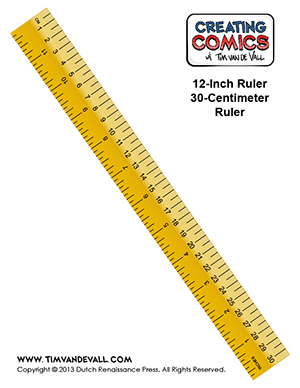# Free Printable Ruler in Inches and Centimeters

Here’s a free printable ruler in inches and centimeters that you can download and print out. Click on any of the ruler thumbnails below to open up the printable ruler pdf. When you print this, do not press “scale to fit” since this will distort the size of the ruler. If you’re printing on 8.5 x 11 paper, the ruler will fit on the paper without getting cut off at the margins. There are several rulers available, including and inches ruler, a centimeter ruler, and an inches and centimeter ruler.## About the Metric System

Here’s some useful facts about the Metric System:

1. The Metric System was introduced by France in 1799.
2. The metric system is also known as the “International System of Units,” of “SI”
3. The fundamental unit of length in the International System of Units is the Meter. (Metre)
4. Since 1983 the meter has been defined as “The length of the path traveled by light in vacuum during a time interval of 1/299,792,458 of a second.)
5. One centimeter = 0.393701 inch (in.)
6. One inch = 2.54 centimeters (cm)
7. The Metric System is used by all nations of the world except Burma, Liberia and the United States.
8. In the United States the metric system is widely used in the areas of science, military, and industry.
9. A centimeter = 1/100th of a meter
10. The circumference of the Earth at the equator is about 40,075 kilometers.

## About the United States Customary Units

Here’s some useful facts about the United States System of Measurement:

1. The US System of units is derived from English units, as system that has its roots in Roman and Anglo-Saxon units.
2. Only the United States, Liberia, and Burma use the United States Customary units.
3. The basic units of measurement in the USA are the inch, foot, yard and mile.
4. One inch = 2.54 centimeters (cm)
5. One centimeter = 0.393701 inch (in.)
6. One foot = 12 inches
7. One yard  = 3 feet (36 inches)
8. One mile = 5,280 feet (1760 yards, 63360 inches)
9. The circumference of the Earth at the equator is about 24,901 miles.
10. While the American Customary units are used in the household, the metric system is widely used in the areas of science, military, and industry.

Source: Wikipedia